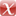# A General Algorithm to Calculate the Inverse Principal p-th Root of Symmetric Positive Definite Matrices

D. Richters, M. Lass, A. Walther, C. Plessl, T. Kühne, Communications in Computational Physics 25 (2019) 564–585.

Journal Article | English
Author
Abstract
We address the general mathematical problem of computing the inverse p-th root of a given matrix in an efficient way. A new method to construct iteration functions that allow calculating arbitrary p-th roots and their inverses of symmetric positive definite matrices is presented. We show that the order of convergence is at least quadratic and that adaptively adjusting a parameter q always leads to an even faster convergence. In this way, a better performance than with previously known iteration schemes is achieved. The efficiency of the iterative functions is demonstrated for various matrices with different densities, condition numbers and spectral radii.
Publishing Year
Journal Title
Communications in Computational Physics
Volume
25
Issue
2
Page
564-585
LibreCat-ID

### Cite this

Richters D, Lass M, Walther A, Plessl C, Kühne T. A General Algorithm to Calculate the Inverse Principal p-th Root of Symmetric Positive Definite Matrices. Communications in Computational Physics. 2019;25(2):564-585. doi:10.4208/cicp.OA-2018-0053
Richters, D., Lass, M., Walther, A., Plessl, C., & Kühne, T. (2019). A General Algorithm to Calculate the Inverse Principal p-th Root of Symmetric Positive Definite Matrices. Communications in Computational Physics, 25(2), 564–585. https://doi.org/10.4208/cicp.OA-2018-0053
@article{Richters_Lass_Walther_Plessl_Kühne_2019, title={A General Algorithm to Calculate the Inverse Principal p-th Root of Symmetric Positive Definite Matrices}, volume={25}, DOI={10.4208/cicp.OA-2018-0053}, number={2}, journal={Communications in Computational Physics}, publisher={Global Science Press}, author={Richters, Dorothee and Lass, Michael and Walther, Andrea and Plessl, Christian and Kühne, Thomas}, year={2019}, pages={564–585} }
Richters, Dorothee, Michael Lass, Andrea Walther, Christian Plessl, and Thomas Kühne. “A General Algorithm to Calculate the Inverse Principal P-Th Root of Symmetric Positive Definite Matrices.” Communications in Computational Physics 25, no. 2 (2019): 564–85. https://doi.org/10.4208/cicp.OA-2018-0053.
D. Richters, M. Lass, A. Walther, C. Plessl, and T. Kühne, “A General Algorithm to Calculate the Inverse Principal p-th Root of Symmetric Positive Definite Matrices,” Communications in Computational Physics, vol. 25, no. 2, pp. 564–585, 2019.
Richters, Dorothee, et al. “A General Algorithm to Calculate the Inverse Principal P-Th Root of Symmetric Positive Definite Matrices.” Communications in Computational Physics, vol. 25, no. 2, Global Science Press, 2019, pp. 564–85, doi:10.4208/cicp.OA-2018-0053.

### Export

Marked Publications

Open Data LibreCat

### SourcesarXiv 1703.02456